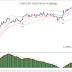## Top Ad unit 728 × 90

Catch the trend forex scalping is a simple trading system. The system has a high accuracy rate and TP can be hit most of the time. This is a trend following trading system.
Time frame 1 min or higher.
Currency pairs: major with low spreads.
Forex Indicators.
Exponential moving average 25 period, high;
Exponential moving average 25 period, low;
Exponential moving average 3 period, close;
Parabolic SAR (0.005 – 0.2),
MACD histogram (24, 48, 12) as filter.
Price close above the channel.
Parabolic SAR below the price.
Exponential moving average 3 period, close above the channel.
MACD histogram above zero level.
Sell
Price close below the channel.
Parabolic SAR above the price.
Exponential moving average 3 period, close below the channel.
MACD histogram below zero level.
The Takeprofit and Stoploss
is at your own discretion. These values depends on the pairs on which you are working on. For example on the 5 min chart, TP 10-20 pips or more. Stop loss 1 pip above/below the EMA channel.
In the charts below random trades examples.

Catch the trend forex scalpingReviewed by learn forex trading on June 15, 2017 Rating: 5